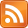The analogue of the derivative for functions whose inputs and outputs are vectors is called the total derivative. The total derivative of a function $f: \mathbb{R}^n \rightarrow \mathbb{R}^m$ is an object that gives you a function for each point in $\mathbb{R}^n$. In other words it is a function $\mathbb{R}^n \rightarrow \mathbb{R}^n \rightarrow \mathbb{R}^m$.

I'll write $D(f)$ for the total derivative of $f$; the function $\mathbb{R}^n \rightarrow \mathbb{R}^n \rightarrow \mathbb{R}^m$. $D(f)(\mathbf{x})$ is what you get when you put in $\mathbf{x}$ — it is a particular function $\mathbb{R}^n \rightarrow \mathbb{R}^m$.

(I wanted to use the more familiar $\frac{d}{d\text{...}}$ notation, but unfortunately it seems to obscure some analogies more than it aids others, because of the need to put in a symbol at the bottom.)

$D(f)(\mathbf{x})$ maps vectors to vectors. I'm going to claim that it "tells you what $f$ does to a very small vector whose tail is placed at $\mathbf{x}$." What does this mean, and how is this a generalization of the derivative?

Think about our old friend $f = x^2$, $\frac{d}{dx} f = 2x$. This means that if you make a very small increase $\epsilon$ to your input at point $x$, your output will increase by $2x\epsilon$. You can view this as a function $\mathbb{R} \rightarrow \mathbb{R} \rightarrow \mathbb{R}$. Given a point $x \in \mathbb{R}$, it gives you a function which maps $\epsilon \rightarrow 2x\epsilon$. It tells you what $f$ does to a very small increase at point $x$.

Now back to the multidimensional case. Adding a very small vector $\mathbf{\epsilon}$ to $\mathbf{x}$ is like making a very small increase to your input, but in multiple dimensions at once. So the total derivative $\mathbb{R}^n \rightarrow \mathbb{R}^n \rightarrow \mathbb{R}^m$ is the analogue of the derivative in the sense that given a point $\mathbf{x} \in \mathbb{R}^n$, it gives you a function which tells you how to map $\mathbf{\epsilon} \rightarrow \text{???}$ for vectors $\mathbf{\epsilon} \in \mathbb{R}^n$ whose tails are placed at $\mathbf{x}$. That's why I said it tells you what $f$ does to a very small vector whose tail is placed at $\mathbf{x}$.

(This post really ought to have some illustrations, but alas I couldn't find any on the Internet, so I'm stuck trying to paint pictures with words.)

An important fact about the total derivative is that it's a linear transformation. With our old friend the derivative, this was so obvious that perhaps it slipped beneath notice. $\epsilon \rightarrow 2x\epsilon$ is linear because it just multiplies the input by some number. All derivatives are linear in this sense.

(Don't get confused: $\frac{d}{dx} x^3 = 3x^2$ isn't a linear function of $x$, but that's the function $\mathbb{R} \rightarrow \mathbb{R} \rightarrow \mathbb{R}$. The thing which we are saying is linear is the function $\mathbb{R} \rightarrow \mathbb{R}$, which is $\epsilon \rightarrow 3x^2 \epsilon$. In introductory calculus classes I've been in, we "squished down" the derivative of a function into $\mathbb{R} \rightarrow \mathbb{R}$ by identifying $\epsilon \rightarrow a\epsilon$ with $a \in \mathbb{R}$. For example, whenever we graphed the derivative of a function. That is all well and good for some things, but I think it is hard to understand the total derivative from that perspective.)

Because there are more dimensions, the total derivative of a function $\mathbb{R}^n \rightarrow \mathbb{R}^m$ can be more complex than "multiply by a number." More things can happen to a very small vector than just scaling; it can be rotated, skewed, etc. One coordinate can be mixed with another. But it is still a linear function of $\mathbf{\epsilon}$. And since it is a linear transformation from $\mathbb{R}^n \rightarrow \mathbb{R}^m$, it can be represented as an $m \times n$ matrix. This is called the Jacobian matrix of the function. We do this by filling out a matrix with every possible partial derivative you could take, organized in a certain way.

For example, take the function $f(\mathbf{x}) = f(x_1, x_2) = \langle x_1^2 + \sin(x_2), x_1x_2 + 10 \rangle$ from $\mathbb{R}^2 \rightarrow \mathbb{R}^2$. We can write down its Jacobian matrix.

$$D(f) = \begin{bmatrix}\frac{\partial f_1}{\partial x_1} & \frac{\partial f_1}{\partial x_2}\\ \frac{\partial f_2}{\partial x_1} & \frac{\partial f_2}{\partial x_2}\end{bmatrix} = \begin{bmatrix}2x_1 & \cos(x_2)\\ x_2 & x_1\end{bmatrix}$$

Fill in a concrete value for $\mathbf{x} = \langle x_1, x_2 \rangle$ and the matrix represents a function $\mathbb{R}^2 \rightarrow \mathbb{R}^2$. It acts on vectors to transform them the way that a very small vector whose tail is at $\mathbf{x}$ would be transformed.

Before I learned about the total derivative, the answer I would give to "how do you generalize derivatives to higher dimensions?" was "with the gradient." The gradient $\nabla f$ is a special case of the total derivative; when the function is $\mathbb{R}^n \rightarrow \mathbb{R}$, the total derivative is a matrix of dimension $1 \times n$ whose transpose can be seen as a column vector in $\mathbb{R}^n$. We take the function $\mathbb{R}^n \rightarrow \mathbb{R}^n \rightarrow \mathbb{R}$ and select just the first part ($\mathbb{R}^n \rightarrow \mathbb{R}^n$) to serve as a vector field. So the gradient is really just the total derivative with some tricks and flips.

The total derivative is the One True Way to think about multi-dimensional derivatives. You can do everything you want with it. It's got a chain rule: $$D(f \circ g)(\mathbf{x}) = D(f)(g(\mathbf{x})) \circ D(g)(\mathbf{x})$$

Note that if you replace $D(f)$ with $f'$, this is almost the same thing as the familiar one-dimensional chain rule $$((f \circ g)(x))' = f'(g(x))g'(x)$$

But mere multiplication in the one-dimensional case has been replaced with composition in the multi-dimensional case. This makes sense because, for one-dimensional linear functions, composition is multiplication. ($(x \rightarrow 5x) \circ (x \rightarrow 2x) = x \rightarrow 10x$.) But when our linear functions get more dimensions, composition becomes more complicated. However, it's still a kind of multiplication — matrix multiplication of the Jacobian matrices!

Here's a table of analogies between the one-dimensional derivative and the total derivative.

d/dx                   |  D
------------------------------------------------
small increase         => add small vector
multiplication         => linear transformation
R -> R -> R            => R^n -> R^n -> R^m
real-valued function   => vector-valued function
f'(g(x))g'(x)          => D(f)(g(x)) o D(g)(x)